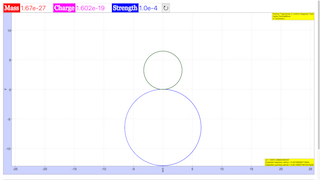Intro Page

Motion of a Charged Particle in a Magnetic Field

Developed by J. D. McDonnell

In this set of exercises, the student will write code to calculate and visualize the trajectories of charged particles under the influence of both uniform and interesting non-uniform magnetic fields, including the earth’s magnetic field.

 Subject Area Electricity & Magnetism Beyond the First Year IPython/Jupyter Notebook Students who complete this set of exercises will develop their understanding of how charged particles respond to magnetic fields (Exercises 1, 2, and 3); be able to describe in pseudo-code how to calculate the trajectory of a charged particle in a magnetic field (Exercise 1); be able to use numerical methods for ordinary differential equations to calculate the particle’s trajectory (Exercises 1, 2, and 3); be able to interpret and describe the computed trajectories (Exercises 1, 2, and 3); and be able to validate numerical solutions against analytical solutions for appropriate test cases (Exercise 1). 120 min
Exercise 1

### EXERCISE 1: MOTION OF A CHARGED PARTICLE IN A UNIFORM MAGNETIC FIELD

Consider a uniform magnetic field, of strength  T, in the -direction. An -particle enters the magnetic field at initial position , with an initial velocity  in the -direction.

• What do you expect the -particle’s trajectory to be shaped like?
• Write the equations of motion for the -particle in the uniform magnetic field. Solve the equations analytically.
• Describe in words (or pseudocode) a procedure to numerically solve these equations of motion for the trajectory of the -particle.
• Now, use your numerical method or a differential equation solver to find a numerical solution to the equations of motion you wrote down. The special case of a uniform magnetic field has an analytical solution, but many cases do not. Validate your code: Does the shape of the -particle’s trajectory match your expectation and your analytical calculation?
• How would your results be different for an  ion that enters the magnetic field? Confirm by running the code with parameters for the  ion with the same initial velocity that the -particle had. Plot the -particle’s trajectory and the negative ion’s trajectory on the same plot.
LorentzForce.ipynb (Exercise 1)

%matplotlib inline

import numpy

import matplotlib.pyplot as mpl

from mpl_toolkits.mplot3d import Axes3D

# Parameters for plot attributes

mpl.rc("xtick", labelsize="large")

mpl.rc("ytick", labelsize="large")

mpl.rc("axes", labelsize="xx-large")

mpl.rc("axes", titlesize="xx-large")

mpl.rc("figure", figsize=(8,8))

### Exercise 1. Uniform Magnetic Field

# define key constants

m_p = 1.67E-27 # mass of proton: kg

qe = 1.602E-19 # charge of proton: C

# now, setting up an alpha particle

m = 4.0*m_p

q = 2.0*qe

QoverM = q/m

dt = 1.0E-8

t = numpy.arange(0.0, 0.002, dt)

rp = numpy.zeros((len(t), 3))

vp = numpy.zeros((len(t), 3))

v0 = numpy.sqrt(2.0*QoverM*10.0)

# negative ion

mn = 1.0 * m_p

qn = -1.0*qe

QoverMn = qn/mn

rn = numpy.zeros((len(t), 3))

vn = numpy.zeros((len(t), 3))

# Strength of Magnetic Field

B0 = 1.0E-4

expected_R = m*v0/(q*B0)

expected_T = 2.0*numpy.pi*expected_R / v0

print("v0 = ", v0)

print("Expected trajectory radius = ", expected_R)

print("Expected cyclotron period = ", expected_T)

# initial condition

rp[0,:] = numpy.array([0.0, 0.0, 0.0])

vp[0,:] = numpy.array([v0, 0.0, 0.0])

# initial condition for negative ion

rn[0,:] = numpy.array([0.0, 0.0, 0.0])

vn[0,:] = numpy.array([v0, 0.0, 0.0])

# Euler time steps

for it in range(0, len(t)-1):

Bp = numpy.array([0.0, 0.0, B0])

Ap = QoverM * numpy.cross(vp[it,:], Bp)

vp[it+1] = vp[it] + dt*Ap

rp[it+1] = rp[it] + dt*vp[it]

An = QoverMn * numpy.cross(vn[it,:], Bp)

vn[it+1] = vn[it] + dt*An

rn[it+1] = rn[it] + dt*vn[it]

# Plot the particle's trajectory, in the xy-plane

mpl.plot(rp[:,0], rp[:,1], label="Alpha particle")

mpl.plot(rn[:,0], rn[:,1], linestyle = "--", label="H$$^-$$ ion")

mpl.xlabel("$$x$$")

mpl.ylabel("$$y$$")

mpl.title("Particle Trajectories in Uniform Magnetic Field")

mpl.legend(fontsize="x-large")

mpl.xlim(-13,13)

mpl.ylim(-13,13)

### Translations

Code Language Translator Run### Software Requirements

SoftwareRequirements

 Android iOS Windows MacOS with best with Chrome Chrome Chrome Chrome support full-screen? Yes. Chrome/Opera No. Firefox/ Samsung Internet Not yet Yes Yes cannot work on some mobile browser that don't understand JavaScript such as..... cannot work on Internet Explorer 9 and below

### CreditsFremont Teng; Loo Kang Wee

[text]

### end faq